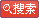# GRE КэС§ҙуИ«

·ўІјКұјдЈә2007-8-30ЎЎОДЧЦҙуРЎЈәҙу ЦР РЎЎЎҙтУЎЈәҙтУЎҙЛОД
GREКэС§»щұҫёЕДоЧЬҪб

ҝјКФИХЖЪЈә2001-05-11 ЎЎ·ўІјКұјдЈә2001-5-11 11:44:00
ЧчХЯЈәlaurry ЎЎЎЎfrom : www.gter.net

Т»ЎЈКэС§»щұҫёЕДо

Т»¶СКэЦРіцПЦЖөВКЧоёЯөДТ»ёц»тјёёцКэ
e.g. mode of 1,1,1,2,3,0,0,0,5 is 1 and 0

Т»¶СКэЦРЧоҙуәНЧоРЎКэЦ®Іо
e.g. range of 1,1,2,3,5 is 5-1=4

geometric mean ЈЁјёәОЖҪҫщКэЈ© nёцКэЦ®»эөДnҙО·Ҫёщ

e.g. median of 1,7,4,9,2,2,2,2,2,5,8 is 2
median of 1,7,4,9,2,5 is (5+7)/2=6

Т»¶СКэЦРЈ¬ГҝёцКэУлЖҪҫщКэөДІоөДҫш¶ФЦөЦ®әНЈ¬іэТФХв¶СКэөДёцКэ(n)
e.g. standard error of 0,2,5,7,6 is:
(|0-4|+|2-4|+|5-4|+|7-4|+|6-4|)/5=2.4

6ЎЈstandard variation
Т»¶СКэЦРЈ¬ГҝёцКэУлЖҪҫщКэЦ®ІоөДЖҪ·ҪЦ®әНЈ¬ФЩіэТФn
e.g. standard variation of 0,2,5,7,6 is: s
_ 2 2 2 2 2_
|_(0-4) +(2-4)+(5-4)+(7-4)+(6-4)_|/5=6.8

7ЎЈstandard deviation
ҫНКЗstandard variationөДЖҪ·Ҫёщ
ұкЧј·ҪІоөД№«КҪЈәd^2=[(a1-a)^2+(a2-a)^2+....+(an-a)^2 ]/n
d ОӘұкЧј·ҪІо

8. ИэҪЗРО УаРю¶ЁАнC^2=A^2+B^2-2ABCOSt tОӘABБҪМхПЯјдөДјРҪЗ

9. Y=k1X+B1,Y=k2X+B2Ј¬БҪПЯҙ№ЦұөДМхјюОӘK1K2=(-1)

10. ИэөДұ¶КэөДМШөгЈәЛщУРО»КэЦ®әНҝЙұ»3Хыіэ

11. NөДҪЧіЛ№«КҪ:
N!=1*2*3*....(N-2)*(N-1)*N
ЗТ№ж¶Ё0!=1
АэИз
8!=1*2*3*4*5*6*7*8

12. КмПӨТ»ПВёщәЕ2Ўў3Ўў5өДЦө
sqrt(2)=1.414
sqrt(3)=1.732
sqrt(5)=2.236

13. ...2/3 as many A as B: A=2/3*B
...twice as many... A as B: A=2*B

14. a if only b: b->a

15. КэС§іЈУГКхУп
THE THIRD POWERКЗИэҙО·ҪөДТвЛј
2^5=the fifth power of 2
abscissa әбЧшұк
ordinate ЧЭЧшұк
coordinate Чшұк
slope РұВК
intercede ҪШҫа(УРХэёәЦ®·Ц)
an=an+(n-1)d s=1/2(a1+an)
common divisor №«ФјКэ
common factor №«ТтЧУ
least common multiple ЧоРЎ№«ұ¶Кэ
composite numbe әПКэ
prime factor ЦКТтЧУ
prime number ЦККэ
factor ТтКэ
consecutive integer Б¬РшөДХыКэ
set јҜәП
sequence КэБР
tenths' digit К®·ЦО»
tenth К®·ЦО»
units' digit ёцО»
whole number ХыКэ
3-digit number ИэО»Кэ
denominator ·ЦДё
numerator ·ЦЧУ
dividend ұ»іэКэ
divided evenly ұ»Хыіэ
divisible ҝЙХыіэөД
divisor іэКэ
quotient ЙМ
remainder УаКэ
round ЛДЙбОеИл
fraction·ЦКэ
geometric progression өИұИКэБР
improper fraction јЩ·ЦКэ
proper fraction Хж·ЦКэ
increase by ФцјУБЛ
increase to ФцјУөҪ
integer ХыКэ
in terms of ..УГЎЈЎЈұнҙп
irrational ОЮАсКэ
multiplier іЛКэ
multiple ұ¶Кэ
multiply іЛ
product іЛ»э
natural number ЧФИ»Кэ
per capita ГҝИЛ
mark up ХЗјЫ
mark down ҪөјЫ
margin АыИу
depreciation ХЫҫЙ
compoud interest ёҙАы
arm ЦұҪЗИэҪЗРОөД№Й
hypotenuse ЦұҪЗИэҪЗРОРұұЯ
lag ЦұҪЗИэҪЗРОөД№Й
median of a triangle ИэҪЗРОЦРПЯ
intersect ПаҪ»
exterior angle НвҪЗ
interior angle ДЪҪЗ
complementary angles УаҪЗ
supplementary angles І№ҪЗ
vertex angle ¶ҘҪЗ
vertical angle ¶Ф¶ҘҪЗ
angle bisector ҪЗЖҪ·ЦПЯ
equilateral triangle өИұЯИэҪЗРО
isosceles triangle өИСьИэҪЗРО
scalene triangle І»өИұЯИэҪЗРО
congruent И«өИөД
rectangle іӨ·ҪРО
length іӨ
both length БҪёціӨұЯ
width ҝн
rectangle prism іӨ·ҪМе
trapezoid МЭРО
rhombus БвРО
diagonal ¶ФҪЗПЯ
perimeter ЦЬіӨ
segment ПЯ¶О
polygon ¶аұЯРО
regular polygon Хэ¶аұЯРО
parallelogram ЖҪРРЛДұЯРО
-agon -ұЯРО *іЈУГ
tetragon ЛДұЯРО
*pentagon ОеұЯРО
*hexagon БщұЯРО
heptagon ЖЯұЯРО
*octagon °ЛұЯРО
enneagon=nonagon ҫЕұдРО
*decagon К®ұдРО
hendecagon=undecagon К®Т»ұЯРО
dodecagon К®¶юұЯРО
quindecagon К®ОеұЯРО
chord ПТ
circumscribe НвЗРЈ¬НвҪУ
inscribe ДЪЗРЈ¬ДЪҪУ
concentric circle Н¬РДФІ
-hedron -ГжМе
hexahedron БщГжМе
volume Ме»э
pyramid ҪЗЧ¶
cube Бў·ҪКэ/Бў·ҪМе
cylinde rФІЦщМе
sphere ЗтМе
NҪЗРОДЪҪЗәН =(n-2)*180

ЕЕБР(permutation):
ҙУNёц¶«¶«(УРЗшұр)ЦРІ»ЦШёҙ(јҙИЎНкәуІ»ФЩИЎ)ИЎіцMёцІўЧчЕЕБР,№ІУРјёЦЦ·Ҫ·Ё
P(M,N)=N!/(N-M)!=N*ЎӯЎӯ..*(N-M+1)

АэИзҙУ1-5ЦРИЎіц3ёцКэІ»ЦШёҙ,ОКДЬЧйіЙјёёцИэО»Кэ
P(3,5)=5!/(5-3)!
=5!/2!
=5*4*3*2*1/(2*1)=5*4*3=60
ТІҝЙТФХвСщПлҙУОеёцКэЦРИЎіцИэёц·ЕИэёц№М¶ЁО»ЦГ
ДЗД·өЪТ»ёцО»ЦГҝЙТФ·ЕОеёцКэЦРИОТ»Т»ёц,ЛщТФУР5ЦЦҝЙДЬСЎ·Ё
..¶ю.. УаПВЛДёцКэЦРИОТ»ёц,....4.....
Иэ... 3....
ЛщТФЧЬ№ІөДЕЕБРОӘ5*4*3=60
Н¬АнҝЙЦӘИз№ыҝЙТФЦШёҙСЎ(јҙИЎНкәуҝЙФЩИЎ),ЧЬ№ІөДЕЕБРКЗ5*5*5=125

ЧйәП(combination):
ҙУNёц¶«¶«(ҝЙТФОЮЗшұр)ЦРІ»ЦШёҙ(јҙИЎНкәуІ»ФЩИЎ)ИЎіцMёц(І»ЧчЕЕБР,јҙІ»№ЬИЎ
өГҙОРтПИәу),№ІУРјёЦЦ·Ҫ·Ё
C(M,N)=P(M,N)/P(M,M)=N!/(M-N)!/M!
C(3,5)=P(3,5)/P(3,3)=5!/2!/3!=5*4*3/(1*2*3)=10

ҝЙТФХвСщАнҪвЈәЧйәПУлЕЕБРөДЗшұрҫНФЪУЪИЎіцөДMёцЧчІ»ЧчЕЕБР-јҙMөДИ«ЕЕБРP
(M,M)=M!Ј¬
ЛщТФC(M,N)*P(M,M)=P(M,N),УЙҙЛҝЙөГЧйәП№«КҪ

РФЦК:C(M,N)=C( (N-M), N )
јҙC(3,5)=C( (5-2), 5 )=C(2,5) = 5!/3!/2!=10

ёЕВКP=ВъЧгДіёцМхјюөДЛщУРҝЙДЬЗйҝцКэБҝ/ЛщУРҝЙДЬЗйҝцКэБҝ
Sorry,ОТГ»УГКхУп
РФЦК
0<=P<=1

a1,a2ОӘБҪБҪІ»ПаИЭөДКВјюЈЁјҙ·ўЙъБЛa1Ј¬ҫНІ»»б·ўЙъa2)
P(a1»тa2)=P(a1)+P(a2)
АэИз
ФтТ»јюКВ·ўЙъөДёЕВК=1 - Т»јюКВІ»·ўЙъөДёЕВКЎЈЎЈЎЈЎЈЎЈЎЈЎЈЎЈЎЈЎЈЎЈ№«КҪ1

АнҪвійПуөДёЕВКЧоәГУГјҜәПөДёЕДоАҙҪІЈ¬·сФтҪбәПҫЯМеМеәГАнҪвРҙ
a1,a2І»КЗБҪБҪІ»ПаИЭөДКВјюЈ¬·ЦұрУГјҜәПAәНјҜәПBАҙұнКҫ
јҜәПAУлјҜәПBөДІўјҜЈ¬ұнКҫОӘA U B ЈЁa1·ўЙъ»тa2·ўЙъЈ©
Фт
PЈЁA U BЈ©= P(A)+P(B)-P(A*B)ЎЈЎЈЎЈЎЈЎЈЎЈЎЈЎЈЎЈЎЈЎЈЎЈЎЈЎЈЎЈЎЈЎЈ№«КҪ2

»№УРҫНКЗМхјюёЕВКЈә
ҝјВЗөДКЗКВјюAТС·ўЙъөДМхјюПВКВјюB·ўЙъөДёЕВК
ОӘКВјюAТС·ўЙъөДМхјюПВКВјюB·ўЙъөДёЕВК
ҫНКЗAУлBН¬Кұ·ўЙъУлA·ўЙъөДёЕВКұИ

АэИз
ФЪE·ўЙъөДЗйҝцПВЈ¬F·ўЙъөДёЕВКОӘ0.45Ј¬ОКEІ»·ўЙъөДЗйҝцПВЈ¬F·ўЙъөДёЕВКУл
0.55ұИҙуРЎ
ТтОӘ!E=1-E
P(!E)=1-P(E) јыЗ°№«КҪ1
P(E+!E)=P(1)=1
ОКP(F|!E)=P(F*!E)/P(!E)
=P(F*(1-E))/P(1-E)
=P(F-F*E)/(P(1)-P(E))
=(P(F)-P(F*E))/(1-P(E)).....МмКйТ»°г,ҝЙТФІ»ҝҙЈ¬№ШјьАнҪвПВГжөДНј
__________________________________________
| ___________ |
| | (~~\~~~~~~~~~Ј© |
| | F( \E Ј© |
| | ( F*E/ Ј© |
| |________(__/ Ј© |
| ~~~~~~~~~~~~ |
|_________________________________________|

ОКEІ»·ўЙъөДЗйҝцПВЈ¬F·ўЙъөДёЕВК
УЙУЪЧЬГж»эУлEЈ¬FёчЧФөДұИАэІ»ЦӘЈ¬ТтҙЛЦөІ»¶Ё

Йи
P(F)=F·ўЙъөДёЕВК
P(E)=E·ўЙъөДёЕВК
P(!E)=EІ»·ўЙъөДёЕВК
P(F|E)=ФЪE·ўЙъөДЗйҝцПВЈ¬F·ўЙъөДёЕВК
P(F|!E)=EІ»·ўЙъөДЗйҝцПВЈ¬F·ўЙъөДёЕВК
P(F,E)=F,EН¬Кұ·ўЙъөДёЕВК
P(F,!E)=F·ўЙъЗТEІ»·ўЙъөДёЕВК

ТтОӘ
P(F)=P(F,E)+P(F,!E)
=P(F|E)*P(E)+P(F|!E)*P(!E)
=P(F|E)*P(E)+P(F|!E)*(1-P(E))
ЛщТФ
P(F|!E)=[P(F)-P(F|E)*P(E)]/(1-P(E))
ЖдЦРP(F|E)=0.45

СЎD.
ХвМвКЗМхјюёЕВКөДјЖЛгЈ¬Из№ыУГ»ӯНјөД·Ҫ·Ё¶ЁРФ·ЦОцТӘИЭТЧөГ¶аЎЈ

ҫИГьИэЧЕ
1ЎЈҙъКэ·Ё
2ЎЈЗоҫЩ·Ё
·ЦұрҫЩјёёцМШАэЈ¬І»·БҙУЧојтөҘөДҫЩЖрЈ¬И»әуЧЬҪбТ»ПВ№жВЙ
3ЎЈФІХы·Ё
¶Фё¶јЖЛгёҙФУөДНјұнМвЈ¬І»·БЛДЙбОеИлЙбИҘБгН·Ј¬ЛгНкәуҝҙёъДЗёцҙр°ёЧоҪУҪьјҙ
ҝЙ

(2001-05-12)

djli 2001-5-12 8:51:35 www.gter.net

¶ФQuartileөДЛөГчЈә
өЪ2ёцQuartileКөјКОӘНЁіЈЛщЛөөДЦР·ЦО»КэЈЁЦРКэЎў¶ю·ЦО»·ЦЎўЦРО»КэЈә
ОТПлҙујТіэБЛ¶Ф1stЎў3rd QuartileІ»БЛҪвНвЈ¬¶ФЖдЛыјёёц
НіјЖБҝөДЗу·Ё¶јКЗұИҪПКмПӨөДБЛЈ¬¶шЗу1stЎў3rdКЗұИҪП
Вй·іөДЈ¬ПВГжТФЗу1rdОӘАэ:
1.ЙиРтБРОӘ{5}Ј¬Ц»УРТ»ёцСщұҫФтЈә(1-1)/4 ЙМ0Ј¬УаКэ0
1st=өЪ1ёцКэ*4/4+өЪ2ёцКэ*0/4=5
2.ЙиРтБРОӘ{1,4}Ј¬УРБҪёцСщұҫФтЈә(2-1)/4 ЙМ0Ј¬УаКэ1
1st=өЪ1ёцКэ*3/4+өЪ2ёцКэ*1/4=1.75
3.ЙиРтБРОӘ{1,5,7}Ј¬УРИэёцСщұҫФтЈә(3-1)/4 ЙМ0Ј¬УаКэ2
1st=өЪ1ёцКэ*2/4+өЪ2ёцКэ*2/4=3
4.ЙиРтБРОӘ{1,3,6,10}Ј¬ЛДёцСщұҫЈә(4-1)/4 ЙМ0Ј¬УаКэ3
1st=өЪ1ёцКэ*1/4+өЪ2ёцКэ*3/4=2.5
5.ЖдЛыАаНЖЈЎ
ФЩУГ1rdөД№«КҪјҙҝЙЗуөГЈә
1.РтБР{5}Ј¬3rd=5
2.{4,1}Ј¬3rd=4*3/4+1*1/4=3.25
3.{7,5,1}Ј¬3rd=7*2/4+5*2/4=6
4.{10,6,3,1}Ј¬3rd=10*1/4+6*3/4=74=64.{10,6,3,1}Ј¬3rd=10*1/4+6*3/4=7

¶ЁАн:
1. ХэХыКэnУРЖжКэёцТтЧУ,ФтnОӘНкИ«ЖҪ·ҪКэ
2. ТтЧУёцКэЗуҪв№«КҪ:Ҫ«ХыКэn·ЦҪвОӘЦКТтЧУіЛ»эРОКҪ,И»әуҪ«ГҝёцЦКТтЧУөДГЭ·Ц
ұрјУТ»ПаіЛ.eg. 200=2*2*2 * 5*5 ТтЧУёцКэ=(3+1)(2+1)=12ёц
3.ДЬұ»8ХыіэөДКэәуИэО»өДәНДЬұ»8Хыіэ;ДЬұ»9ХыіэөДКэёчО»КэөДәНДЬұ»9Хыіэ.
4.¶аұЯРОДЪҪЗәН=(n-2)x180
5.БвРОГж»э=1/2 x ¶ФҪЗПЯіЛ»э

ЦЭіӨ№ӨЧКМв(Stem-and-Leaf)ҪвҙрЈЁАҙЧФГЧ№ъ) teddybear 2001-08-14
15:06:22 (from taisha)
ХвұҫАҙКЗ»ШУҰПВГжТ»ёцМыЧУөДЈ¬Ҫб№ыРБРБҝаҝаРҙБЛ°лМмҫ№И»Г»ДЬМыЙПЈ¬ЛщТФЦ»ДЬ
ЦШРВРҙ№эЈ¬ЧчОӘРВМыЈ¬ПЈНы¶ФҙујТУРЛщ°пЦъЎЈ
-------------------------------------------------
Stem-and-Leaf ( 50ЦЬіӨ№ӨЧКМв)
ХвКЗОТФЪГЧ№ъҙу¶юНіјЖҝОЙПС§өДЈ¬Stem and Leaf әНHistogramТ»СщЈ¬¶јКЗНіјЖ
С§УГөДТ»ЦЦcollect and represent КэҫЭөД·Ҫ·ЁЎЈ Stem and LeafөДёЕДоЖдКөәЬ
јтөҘЈ¬УГУпСФІ»М«әГҪвКНЈ¬ОТ»№КЗҫЩАэәГБЛЎЈ

0| 1 2 2 4
1| 2 5 8
2| 0 3 3 4 7
5| 1 9
Stem (unit) = 10
Leaf (unit) = 1

·ЦОцИзПВЈә ЧоЧуұЯөДТ»КъРР 0, 1, 2, 5ҪРЧцStem, ¶шУТұЯКЈПВөДҫНКЗLeaf
(leaves)

    ПВТ»Ті

ОДХВЈәЎ°GRE КэС§ҙуИ«ЎұХэОДНк
ИИөгОДХВ
ФЪПЯЧЙСҜ
ЎЎ